Courses

# Examples - Centre of Gravity and Centroid Mechanical Engineering Notes | EduRev

## Mechanical Engineering : Examples - Centre of Gravity and Centroid Mechanical Engineering Notes | EduRev

The document Examples - Centre of Gravity and Centroid Mechanical Engineering Notes | EduRev is a part of the Mechanical Engineering Course Engineering Mechanics - Notes, Videos, MCQs & PPTs.
All you need of Mechanical Engineering at this link: Mechanical Engineering

Three particles (point masses) of mass 2 kg, 3 kg, and 3 kg, are welded to a straight massless rod as shown in the figure. Find the location of the center of mass of the assembly.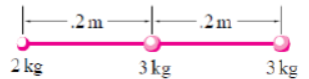Solution Let us select the first mass, m1 D 2 kg, to be at the origin of our coordinate system with the x-axis along the rod. Since all the three masses lie on the x-axis, the center of mass will also lie on this axis. Let the center of mass be located at xcm on the x-axis. Then,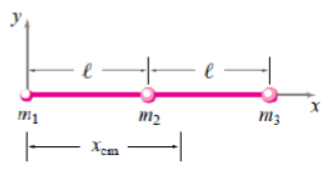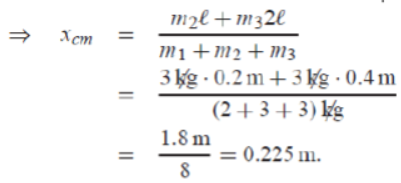Alternatively, we could find the center of mass by first replacing the two 3 kg masses with a single 6 kg mass located in the middle of the two masses (the center of mass of the two equal masses) and then calculate the value of xcm for a two particle system consisting of the 2 kg mass and the 6 kg mass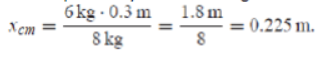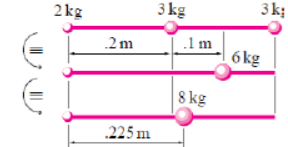Two particles of mass m1 = 1 kg and m2 = 2 kg are located at coordinates (1m, 2m) and (-2m, 5m), respectively, in the xy-plane. Find the location of their center of mass.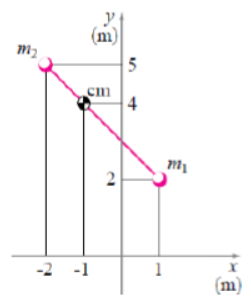Solution Let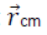be the position vector of the center of mass. Then,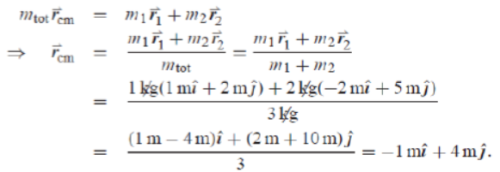Thus the center of mass is located at the coordinates(-1m, 4m).

A structure is made up of three point masses, m1 = 1 kg; m2 = 2 kg and m3 = 3 kg, connected rigidly by massless rods. At the moment of interest, the coordinates of the three masses are (1.25 m, 3 m), (2 m, 2 m), and (0.75 m, 0.5 m), respectively. Find the coordinates of the center of mass of the structure.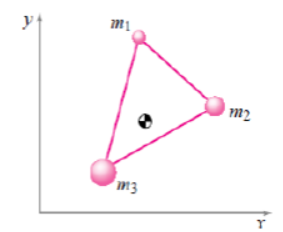Solution Let (xcm , ycm) be the coordinates of the mass-center. Then from the definition of mass-center,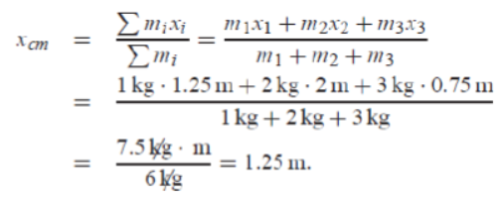Similarly,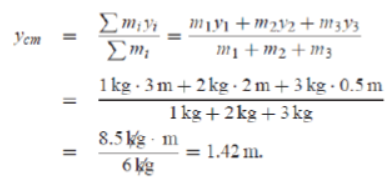Thus the center of mass is located at the coordinates (1.25 m, 1.42 m).

Center of mass of a bent bar: A uniform bar of mass 4 kg is bent in the shape of an asymmetric ’Z’ as shown in the figure. Locate the center of mass of the bar.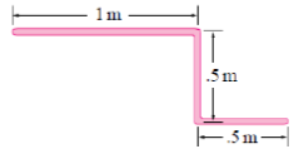Solution. Since the bar is uniform along its length, we can divide it into three straight segments. The mass of each segment is proportional to its length. Therefore, if we let m2 = m3 = m, then m1 = 2m; and m1 + m2 + m3 = 4m = 4 kg which gives m = 1 kg.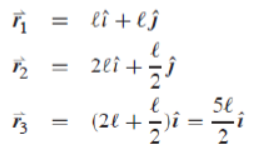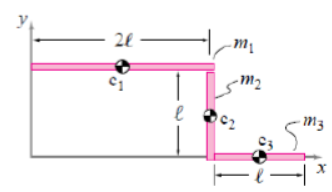So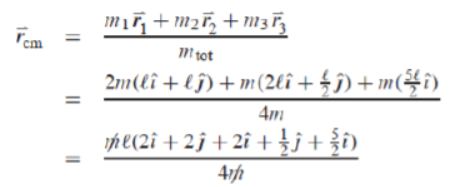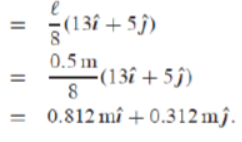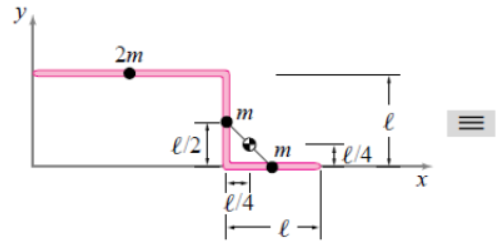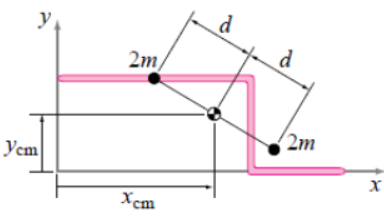Find Centroid of the cross-section or Area in figs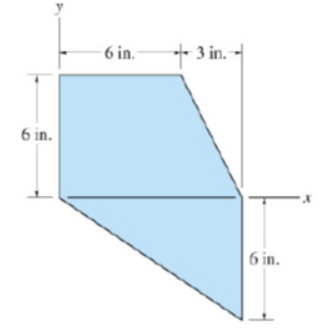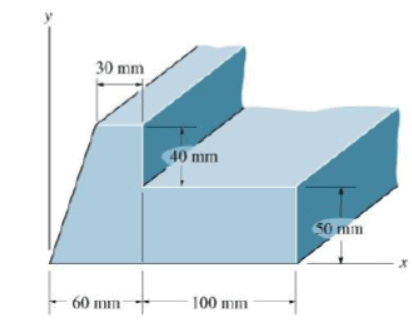Offer running on EduRev: Apply code STAYHOME200 to get INR 200 off on our premium plan EduRev Infinity!

,

,

,

,

,

,

,

,

,

,

,

,

,

,

,

,

,

,

,

,

,

;